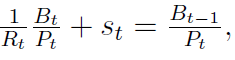# Government Debt in a RBC vs NK Model

Hi

I have a general question about the nature of government debt in a RBC model vs NK model.

Consider a simple government budget constraint in an RBC model:

R_(t-1)B_(t-1)=B_t+T_t where T is taxes. R is short-term nominal rate. T responds to past levels of gov. debt (i.e. T (B_(t-1)). It is thus clear that B_t is predetermined in period t.

The equivalent budget constraint in a NK model would look like this:

R_(t-1)B_(t-1)/pi_t=B_t+T_t where now pi_t is inflation (and thus the budget constraint is written in real terms).

Now, B_t is also a function of pi_t, which is a jump variable. Thus, technically B_t will change in period t because of inflation. Yet, B_t is still technically not a jump variable since agents don’t form expectations over debt. So, is B_t still technically considered predetermined in the NK model, even though its value will change in period t?

Hi sp1589,

It is the real price of the stock of bonds that is affected by current inflation, not the stock of bonds itself. The stock of bonds is predetermined, the real price is not.Indeed, in a standard NK model set-up you have that in the budget constraint

\frac{B_t}{P_t}+\text{other items}=\frac{B_{t-1}}{P_{t-1}}\frac{R_{t-1}}{\pi_t}+\text{other items}

So, given some shock in the economy, that is going to affect inflation (and thus the real price of the bond). In period t, this means that only inflation will adjust in the government’s budget constraint. So, next period (t+1), the stock of bonds will adjust conditional on period t’s inflation (or alternatively interpreted conditional on the change in the real price of the stock). In the current period t, however only inflation adjusts in the gov’s budget constraint as the other variables are predetermined. Is that interpretation correct?

B_{t-1} was a promise to repay 100 Dollars plus whatever the interest rate was. Say R_{t-1}=1.05. Then the government promised to repay 105 Dollars today. That promise cannot be changed anymore at time t, because it was made at t=-1. That’s why B_{t-1} is a predetermined variable as indicated by the timing. B_t itself is not predetermined. If the real value of debt turns out to be very high today, the government may need to issue more debt this period B_t.

Yes, I understand that. I was just confused based on the initial reply and was trying to clarify what was meant. My question is more fundamental.

Consider consumption C_t. That variable is a forward-looking non-predetermined because agents realize that in the event of a shock they will change their expected future consumption E_tC_(t+1) which would also imply an adjustment in their current consumption C_t.

On the other hand, B_t here is not a forward-looking variable. It only changes in period T, because it is a function of another forward looking variable - inflation.

My initial confusion here was how to classify C_t and B_t. They are both non-predetermined in period T, but the fundamental reason for why they adjust is different.

Not really. C_t will also adjust, because other jump variables have moved. After all, the budget constraint needs to be satisfied.

Hi, cmarch.
A quick question. Why did you divide the nominal interest rate by gross inflation?
Leeper and Leith (2016) do not do that on page 30:In your model, you will have real bonds B_t/P_t. That requires you to expand the fraction \frac{B_{t-1}} {P_t}=\frac{B_{t-1}} {P_t}\frac{P_{t-1}} {P_{t-1}}=\frac{B_{t-1}} {P_{t-1}\Pi_t}

1 Like